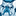# QlikView App Dev

Discussion Board for collaboration related to QlikView App Development.

Announcements
Join us for a live Q&A! September 21, 10 AM ET - Onboarding Fast in Qlik Sense SaaS! REGISTER
cancel
Showing results for
Did you mean:Contributor II

## Rounding off a number- how to actually change it?

I have two fields of numbers I am comparing: one which is calculated (and therefore it has many digits potentially after the decimal), and one which already only has 2 decimal places.

For example, assume my script is as follows:

Sales

Percentage

Sales * Percentage as [Number 1]

[Number 2]

The result for Number 1 might in example be 345.98765

Assume Number 2 for this record is 345.99

I then have a flag calculated along the lines of: if( [Number 1]=[Number 2], 'OK', 'Not OK') as NumberFlag

Currently, the fact pattern above would result in a 'Not OK' for this record because Qlik is comparing the full result of Number 1 to Number 2, and determining that Number 2 is greater. But what I want is for Qlik to first round Number 1 to 345.99 and then compare that rounded value to Number 2, which would return an 'OK' result.

I've tried using the rounding function and the num function as follows:

round((Sales * Percentage),0.01) as [Number 1]

num((Sales * Percentage),'##.##') as [Number 1]

But neither of these seems to actually change the underlying amount. Number 1 will show up in a table as 345.99, but the NumberFlag will be 'Not OK'.

What am I missing here?

2 RepliesMVP

I think this blog will clearly explain the issue to you -> Rounding ErrorsMVP

chk dis

or else numb tab u cn select  Fixed  value to  "2"

Rounding ErrorsCommunity Browser